• Slides: 28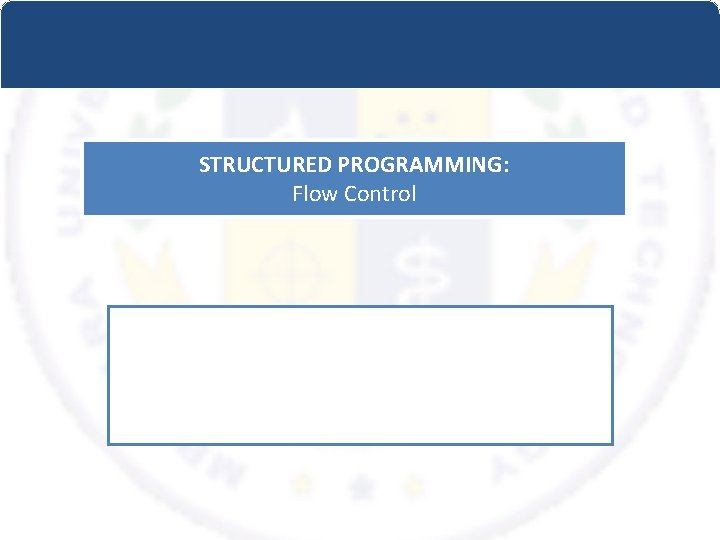STRUCTURED PROGRAMMING: Flow ControlComments • Comments: /* This is a comment */ – Use them! – Comments should explain: • special cases • the use of functions (parameters, return values, purpose) • special tricks or things that are not obvious – explain WHY your code does things the way it does.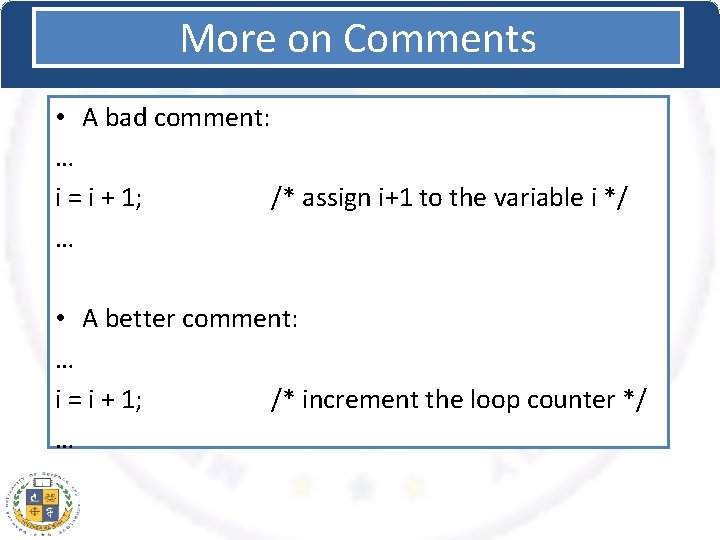More on Comments • A bad comment: … i = i + 1; /* assign i+1 to the variable i */ … • A better comment: … i = i + 1; /* increment the loop counter */ …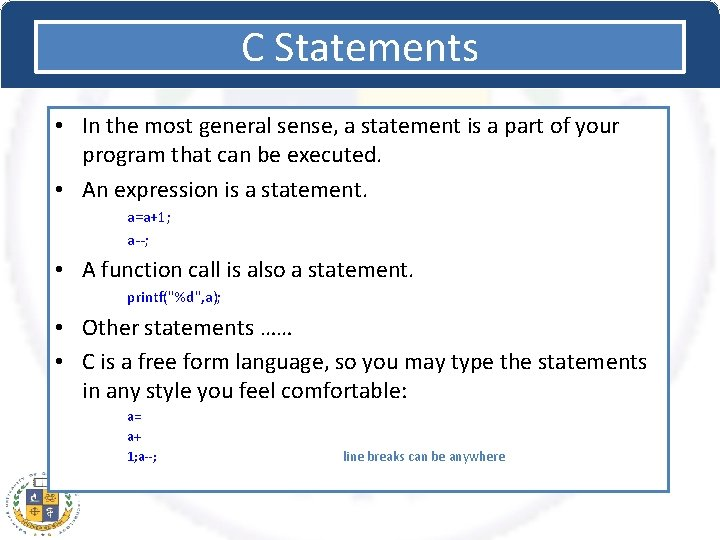C Statements • In the most general sense, a statement is a part of your program that can be executed. • An expression is a statement. a=a+1; a--; • A function call is also a statement. printf("%d", a); • Other statements …… • C is a free form language, so you may type the statements in any style you feel comfortable: a= a+ 1; a--; line breaks can be anywhereCompound Statements • Sequences of statements can be combined into one with {. . . } • Much like Java: { printf ("Hello, "); printf ("world! n"); } • The C compiler treats the collection of these statements like they are a single statement.C Statements Some Suggestions • DO: stay consistent with how you use whitespace • DO: put block braces on their own line. – This makes the code easier to read. • DO: line up block braces so that it is easy to find the beginning and end of a block. • AVOID: spreading a single statement across multiple lines if there is no need. – Try to keep it on one line.SELECTION • The Selection construct means the execution of statement(s) depending upon a condition-test. If a condition evaluates to true, a course-of-action (a set of statements) is followed otherwise another courseof-action (a different set of statements). • This construct(selection construct) is also called decision construct because it helps in making decision about which set-of-statements is to be executed.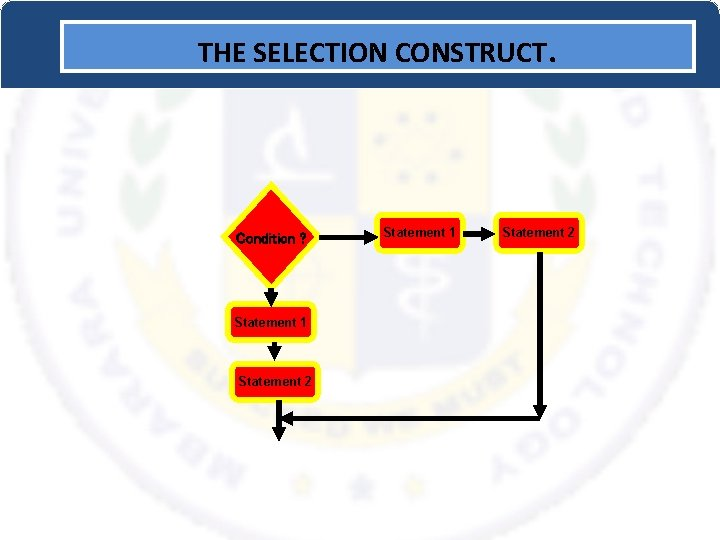THE SELECTION CONSTRUCT. Condition ? Statement 1 Statement 2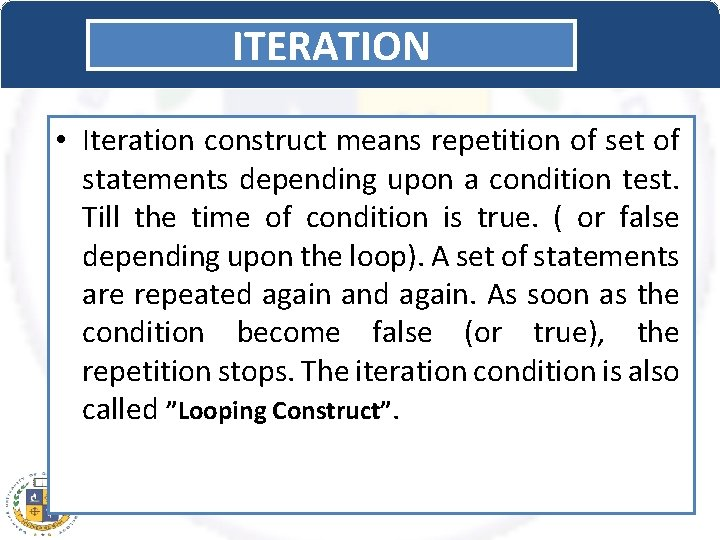ITERATION • Iteration construct means repetition of set of statements depending upon a condition test. Till the time of condition is true. ( or false depending upon the loop). A set of statements are repeated again and again. As soon as the condition become false (or true), the repetition stops. The iteration condition is also called ”Looping Construct”.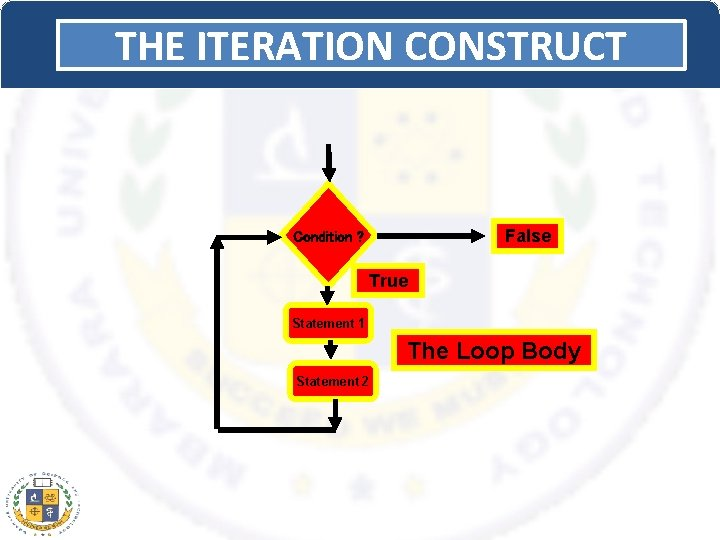THE ITERATION CONSTRUCT False Condition ? True Statement 1 The Loop Body Statement 2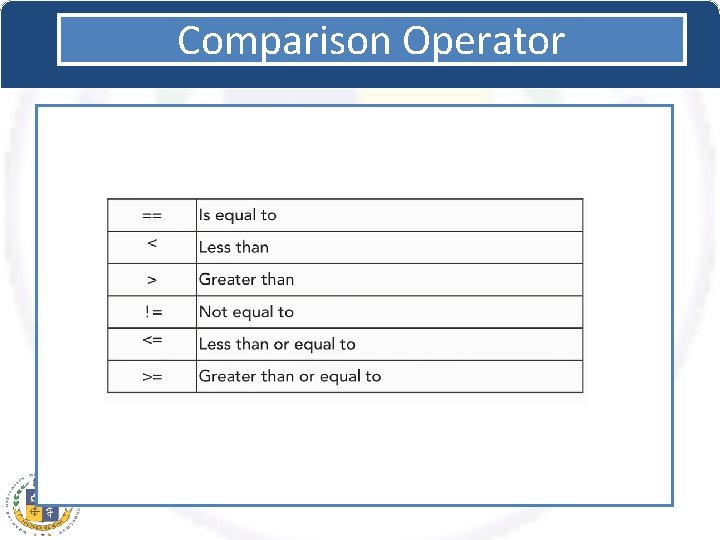Comparison Operator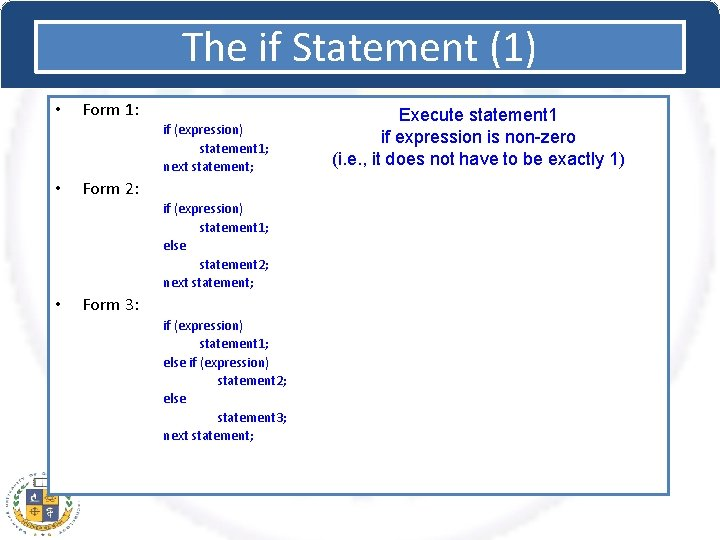The if Statement (1) • Form 1: if (expression) statement 1; next statement; • Form 2: if (expression) statement 1; else statement 2; next statement; • Form 3: if (expression) statement 1; else if (expression) statement 2; else statement 3; next statement; Execute statement 1 if expression is non-zero (i. e. , it does not have to be exactly 1)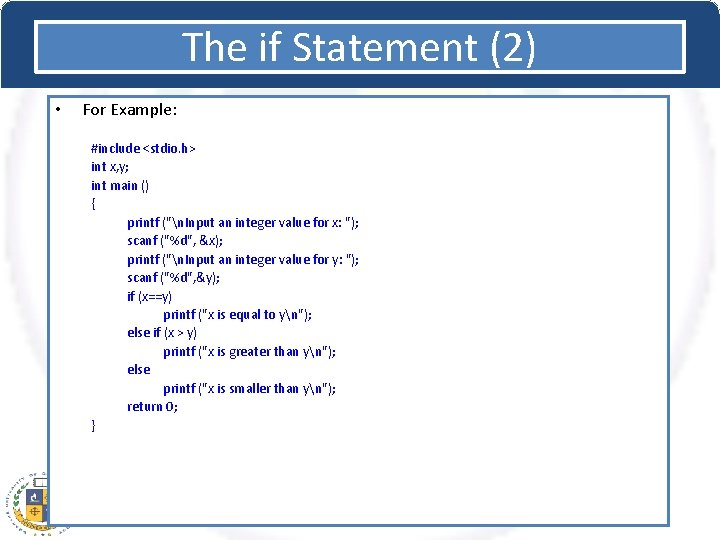The if Statement (2) • For Example: #include <stdio. h> int x, y; int main () { printf ("n. Input an integer value for x: "); scanf ("%d", &x); printf ("n. Input an integer value for y: "); scanf ("%d", &y); if (x==y) printf ("x is equal to yn"); else if (x > y) printf ("x is greater than yn"); else printf ("x is smaller than yn"); return 0; }The for Statement (1) • • The most important looping structure in C. Generic Form: for (initial ; condition ; increment ) statement • • initial, condition, and increment are C expressions. For loops are executed as follows: 1. 2. 3. 4. 5. 6. initial is evaluated. Usually an assignment statement. condition is evaluated. Usually a relational expression. If condition is false (i. e. 0), fall out of the loop (go to step 6. ) If condition is true (i. e. nonzero), execute statement Execute increment and go back to step 2. Next statementThe for Statement (2) /* 4. initialization outside of loop */ count = 1; for ( ; count < 1000; count++) printf("%d ", count); For statement examples #include <stdio. h> int main () { int count, x, y; int ctd; /* 5. very little need be in the for */ count=1; ctd=1; for ( ; ctd; ) { printf("%d ", count); count++; ctd=count<1000; } /* 1. simple counted for loop */ for (count =1; count <=20; count++) printf ("%dn", count); /* 2. for loop counting backwards */ for (count = 100; count >0; count--) { x*=count; printf("count=%d x=%dn", count, x); } /* 6. compound statements for initialization and increment */ for (x=0, y=100; x<y; x++, y--) { printf("%d %dn", x, y); } return 0; /* 3. for loop counting by 5's */ for (count=0; count<1000; count += 5) y=y+count; }The for Statement (3) • Nesting for Statements – for statements (and any other C statement) can go inside the loop of a for statement. – For example: #include <stdio. h> int main( ) { int rows=10, columns=20; int r, c; for ( r=rows ; r>0 ; r--) { for (c = columns; c>0; c--) printf ("X"); printf ("n"); } }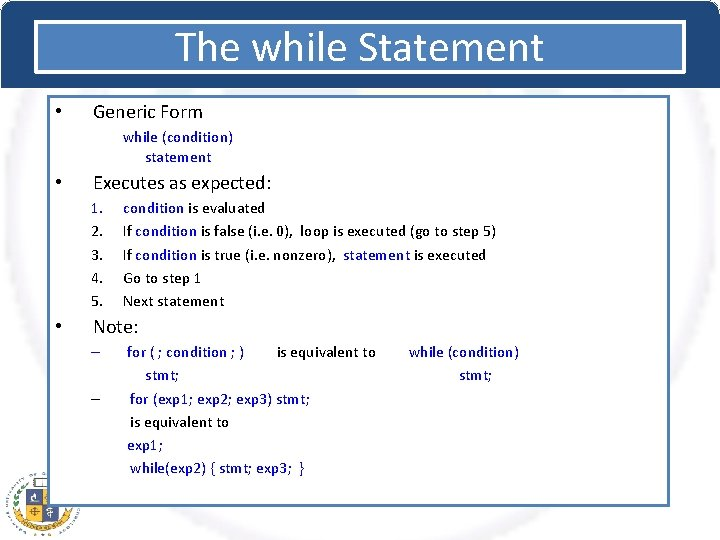The while Statement • Generic Form while (condition) statement • Executes as expected: 1. 2. 3. 4. 5. • condition is evaluated If condition is false (i. e. 0), loop is executed (go to step 5) If condition is true (i. e. nonzero), statement is executed Go to step 1 Next statement Note: – – for ( ; condition ; ) is equivalent to stmt; for (exp 1; exp 2; exp 3) stmt; is equivalent to exp 1; while(exp 2) { stmt; exp 3; } while (condition) stmt;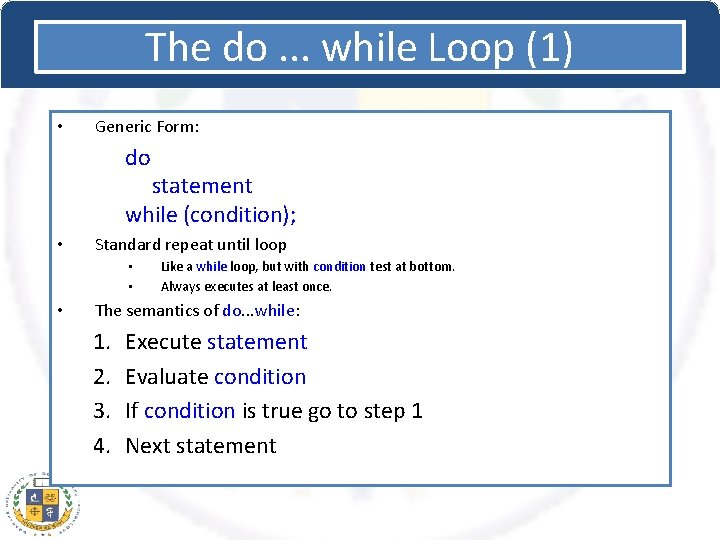The do. . . while Loop (1) • Generic Form: do statement while (condition); • Standard repeat until loop • • • Like a while loop, but with condition test at bottom. Always executes at least once. The semantics of do. . . while: 1. 2. 3. 4. Execute statement Evaluate condition If condition is true go to step 1 Next statement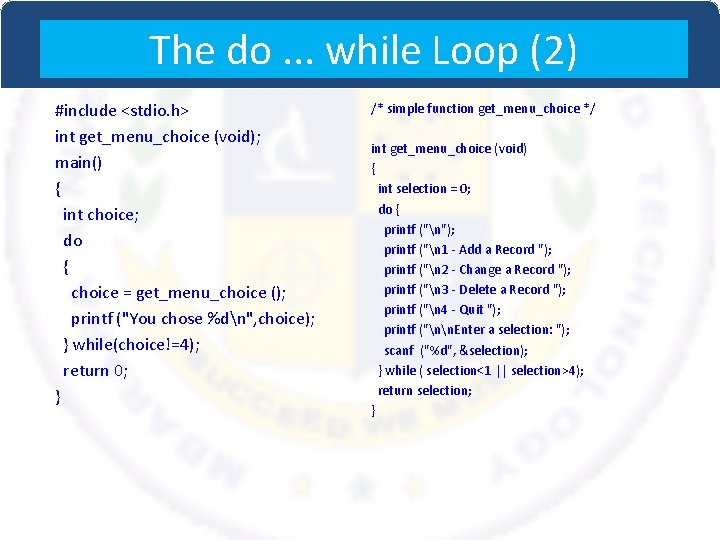The do. . . while Loop (2) #include <stdio. h> int get_menu_choice (void); main() { int choice; do { choice = get_menu_choice (); printf ("You chose %dn", choice); } while(choice!=4); return 0; } /* simple function get_menu_choice */ int get_menu_choice (void) { int selection = 0; do { printf ("n"); printf ("n 1 - Add a Record "); printf ("n 2 - Change a Record "); printf ("n 3 - Delete a Record "); printf ("n 4 - Quit "); printf ("nn. Enter a selection: "); scanf ("%d", &selection); } while ( selection<1 || selection>4); return selection; }break and continue • The flow of control in any loop can be changed through the use of the break and continue commands. • The break command exits the loop immediately. – Useful for stopping on conditions not controlled in the loop condition. – For example: for (x=0; x<10000; x++) { if ( x*x % 5==1) break; . . . do some more work. . . } – Loop terminates if x*x % 5 == 1 • The continue command causes the next iteration of the loop to be started immediately. – For example: for (x=0; x<10000; x++) { if (x*x % 5 == 1) continue; printf( "%d ", 1/ (x*x % 5 – 1) ); } – Don't execute loop when x*x % 5 == 1 (and avoid division by 0)Example: for and break Together const int mycard=3; int guess; for(; ; ) { printf("Guess my card: "); scanf("%d", &guess); if(guess==mycard) { printf("Good guess!n"); break; } else printf("Try again. n"); } The notation for(; ; ) is used to create an infinite for loop. while(1) creates an infinite while loop instead. To get out of an infinite loop like this one, we have to use the break statement.switch Statement • • Switch statement is used to do “multiple choices”. Generic form: switch(expression) { case constant_expr 1 : statements case constant_expr 2 : statements … case constant_exprk : statements default : statements } 1. expression is evaluated. 2. The program jumps to the corresponding constant_expr. 3. All statements after the constant_expr are executed until a break (or goto, return) statement is encountered.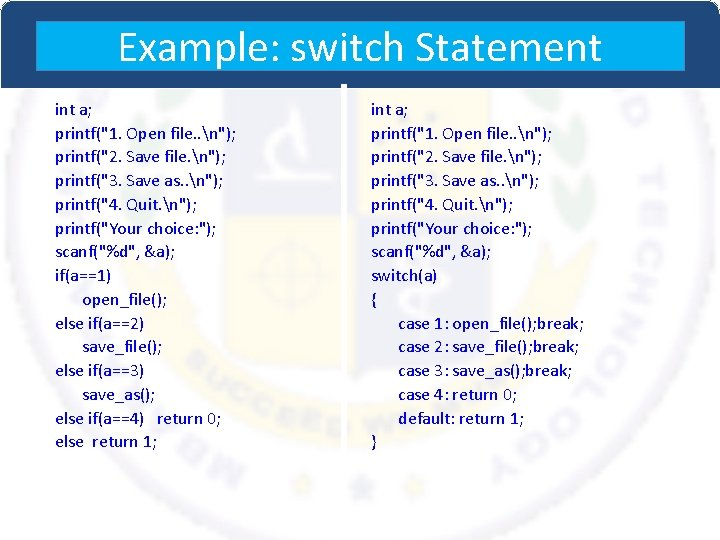Example: switch Statement int a; printf("1. Open file. . n"); printf("2. Save file. n"); printf("3. Save as. . n"); printf("4. Quit. n"); printf("Your choice: "); scanf("%d", &a); if(a==1) open_file(); else if(a==2) save_file(); else if(a==3) save_as(); else if(a==4) return 0; else return 1; int a; printf("1. Open file. . n"); printf("2. Save file. n"); printf("3. Save as. . n"); printf("4. Quit. n"); printf("Your choice: "); scanf("%d", &a); switch(a) { case 1: open_file(); break; case 2: save_file(); break; case 3: save_as(); break; case 4: return 0; default: return 1; }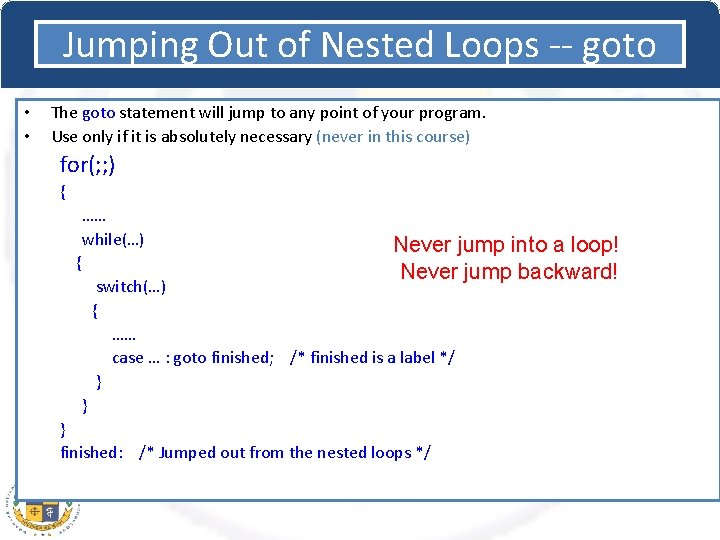Jumping Out of Nested Loops -- goto • • The goto statement will jump to any point of your program. Use only if it is absolutely necessary (never in this course) for(; ; ) { …… while(…) { Never jump into a loop! Never jump backward! switch(…) { …… case … : goto finished; /* finished is a label */ } } } finished: /* Jumped out from the nested loops */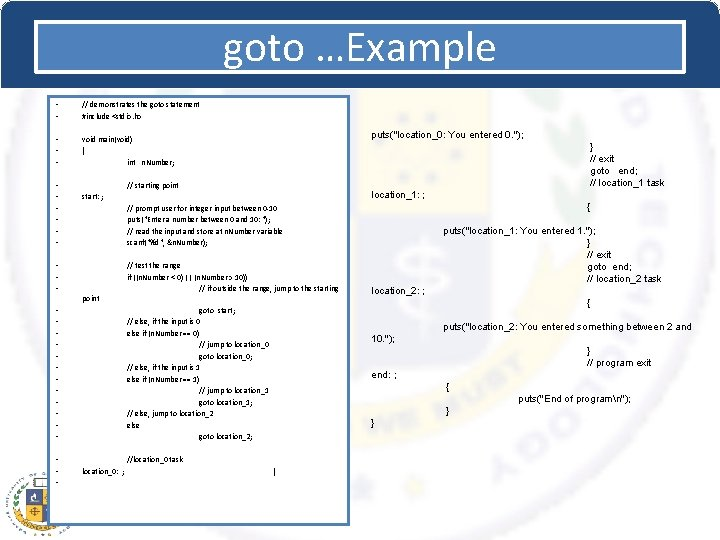goto …Example • • // demonstrates the goto statement #include <stdio. h> • • • void main(void) { int n. Number; • • • } // exit goto end; // location_1 task // starting point location_1: ; start: ; { // prompt user for integer input between 0 -10 puts("Enter a number between 0 and 10: "); // read the input and store at n. Number variable scanf("%d", &n. Number); • • • point • • • • puts("location_0: You entered 0. "); // test the range if ((n. Number < 0) || (n. Number > 10)) // if outside the range, jump to the starting location_2: ; { goto start; // else, if the input is 0 else if (n. Number == 0) // jump to location_0 goto location_0; // else, if the input is 1 else if (n. Number == 1) // jump to location_1 goto location_1; // else, jump to location_2 else goto location_2; puts("location_2: You entered something between 2 and 10. "); } // program exit end: ; { puts("End of programn"); } } //location_0 task location_0: ; puts("location_1: You entered 1. "); } // exit goto end; // location_2 task {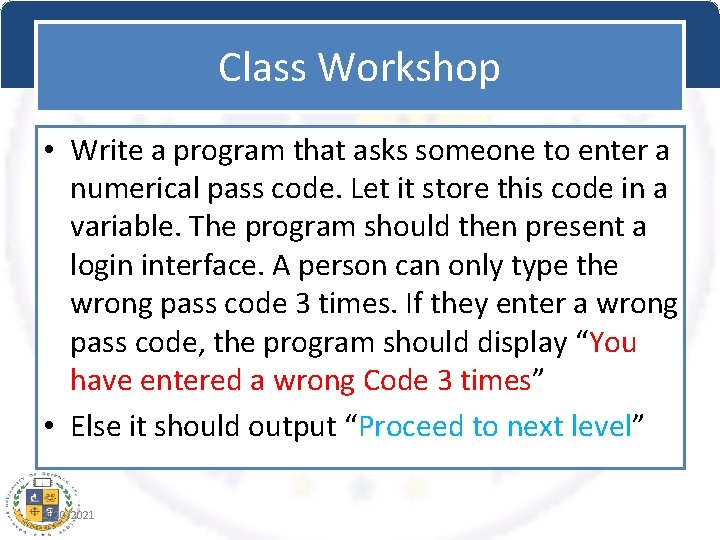Class Workshop • Write a program that asks someone to enter a numerical pass code. Let it store this code in a variable. The program should then present a login interface. A person can only type the wrong pass code 3 times. If they enter a wrong pass code, the program should display “You have entered a wrong Code 3 times” • Else it should output “Proceed to next level” 2/20/2021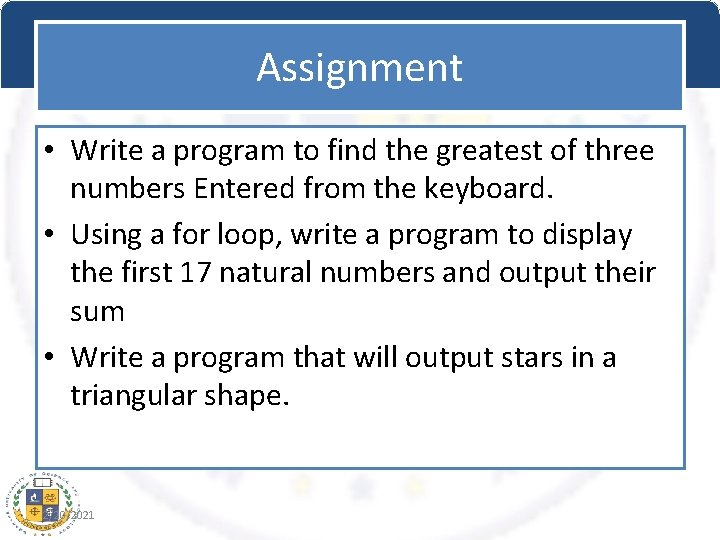Assignment • Write a program to find the greatest of three numbers Entered from the keyboard. • Using a for loop, write a program to display the first 17 natural numbers and output their sum • Write a program that will output stars in a triangular shape. 2/20/2021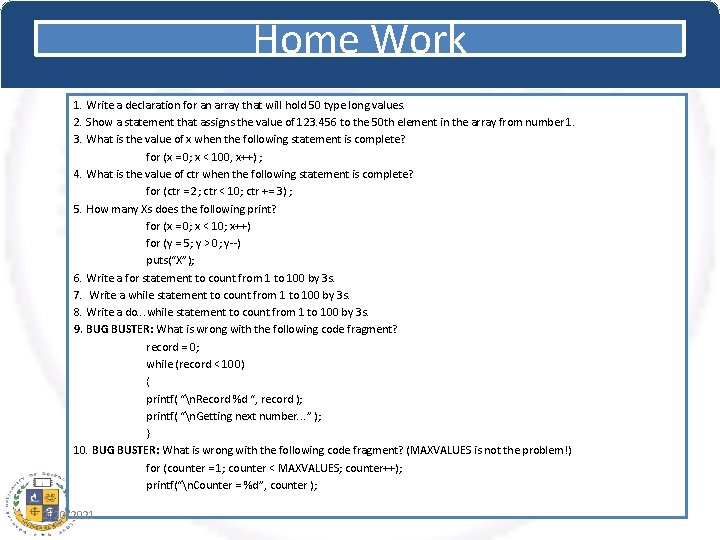Home Work 1. Write a declaration for an array that will hold 50 type long values. 2. Show a statement that assigns the value of 123. 456 to the 50 th element in the array from number 1. 3. What is the value of x when the following statement is complete? for (x = 0; x < 100, x++) ; 4. What is the value of ctr when the following statement is complete? for (ctr = 2; ctr < 10; ctr += 3) ; 5. How many Xs does the following print? for (x = 0; x < 10; x++) for (y = 5; y > 0; y--) puts(“X”); 6. Write a for statement to count from 1 to 100 by 3 s. 7. Write a while statement to count from 1 to 100 by 3 s. 8. Write a do. . . while statement to count from 1 to 100 by 3 s. 9. BUG BUSTER: What is wrong with the following code fragment? record = 0; while (record < 100) { printf( “n. Record %d “, record ); printf( “n. Getting next number. . . ” ); } 10. BUG BUSTER: What is wrong with the following code fragment? (MAXVALUES is not the problem!) for (counter = 1; counter < MAXVALUES; counter++); printf(“n. Counter = %d”, counter ); 2/20/2021